Next: Geometric Based Algorithms Up: Load Balancing for Unstructured Previous: Graph Theory Notation

The static load balancing problem for a mesh based application involves partitioning the mesh into subdomains. The subdomains can then be distributed over the processors and calculation carried out in parallel. Different partitions of the mesh may result in different times to completion for the calculation. It is therefore necessary to examine the quality of the partitioning based on its effect on the application code. There are a number of factors.

The first factor is that of the load balance. The computational work of each processor should be balanced, so that no processor will be waiting for others to complete. Assuming that the computational work per processor is proportional to the number of mesh nodes in the subdomain, then to achieve load balance it is necessary for the number of nodes in each subdomain to be the same.

The second factor is that of the communication cost. When forming the discretized equations on a node of the mesh, the contributions from its nearest neighbor nodes will usually be needed. Depending on the order of the discretization scheme, contributions from more distant neighboring nodes may be necessary. On a parallel computer, accumulating the contributions from nodes that are not on the current processor will incur communication cost. It is known that on distributed memory parallel computers the cost of accessing remote memory is far higher than that of accessing local memory (typically a ratio of between 10 to 1000). It is therefore important to minimize the communication cost.

There are other factors that affect the time to completion of a parallel calculation. Often, to maximize the parallelism, the solver algorithm implemented on a parallel computer is not chosen to be mathematically equivalent to the sequential algorithm. Therefore the number of iterations needed for a parallel code to converge on multi-processors may not be the same as that on a single-processor. This is particularly true when implicit algorithms are used, and the way a mesh is partitioned can affect the rate of convergence considerably. However for now, we will in essence assume that a linear explicit solver is being used and seek to meet two criteria

• Each subdomain should have a roughly equal number of nodes;
• The communication cost is minimized.

It is useful to introduce the idea of a communication graph, and to distinguish the computational mesh from its communication graph. The communication graph characterizes the data dependency of the computation on the mesh. For instance, when constructing a communication graph for the purpose of partitioning a finite element mesh,

If the finite element algorithm has a node based data structure, then computation on each node requires data from neighboring nodes (those nodes which share an edge with this node). Therefore data dependency is along the edges of the mesh, in which case vertices of the communication graph are simply the nodes of the mesh, and the edges of the graph coincide with the edges of the mesh. Alternatively, if the finite element algorithm has an element based data structure, then computation on each element requires data from neighboring elements (those elements which share a face with this element). Therefore the data dependency is across the faces of the mesh. Vertices of the communication graph are now at the centroid of the elements, and two vertices of the graph form an edge if the corresponding elements share a face. Such a graph is called the dual graph of the mesh.
The partitioning of a mesh is usually based on the partitioning of its corresponding communication graph. The number of edges of the communication graph cut by a partitioning is called the number of edges-cut (or edge-cut for short) of the partitioning. The communication cost of a subdomain is a function of its edge-cut as well as the number of neighboring subdomains that share edges with it. In practice the edge-cut of a partitioning is usually used as an important indicator of the quality of the partitioning. Figure 2 shows a mesh of 788 elements for an element based finite element calculation, and the dual graph of the mesh. The partitioning of the dual graph (Figure 2 (right)) into 4 subdomains, using the recursive coordinate bisection (RCB) algorithm described in Section 3.1.1, results in the partitioning of the mesh shown in Figure 2 (left).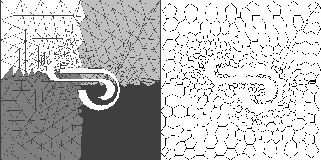When there are only two subdomains or processors, the graph partitioning problem becomes a graph bisection problem, where given a graph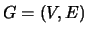with vertices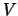and edges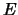, it is required to find a partition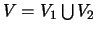such that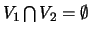,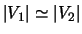and the edge-cut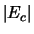: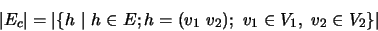is minimized. Here for a set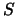, one denotes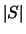as the number of elements in the set.

The graph bisection problem has been studied in the past by many authors (see, e.g., [53,63,11]) in the context of graph theory as well as VLSI circuit layout. Advances in parallel computing hardware and software has renewed interest in the problem. The graph bisection problem is an NP hard problem, so there are no known algorithms that can find the exact solution to the problem in polynomial time. Most of the graph bisection methods therefore seek a good approximation to the optimal partitioning that can be calculated efficiently.

In the following a number of partitioning algorithms are described. Many of them are bisection based. A partitioning for an arbitrary number of processors requires the recursive use of the bisection algorithm (for a number that is not a power of two, the bisection will give two subdomains of different sizes, see ). We shall denote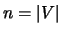the number of vertices of the graph, and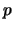the number of processors (subdomains).

SubsectionsNext: Geometric Based Algorithms Up: Load Balancing for Unstructured Previous: Graph Theory Notation

2000-03-21